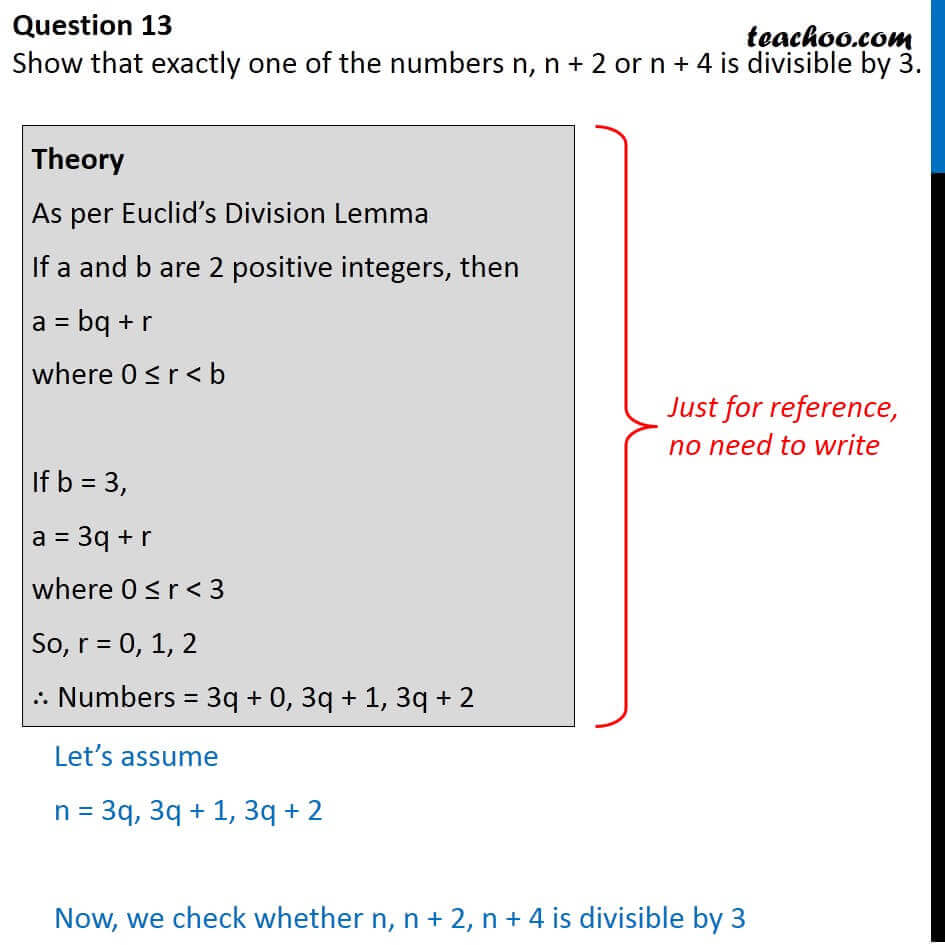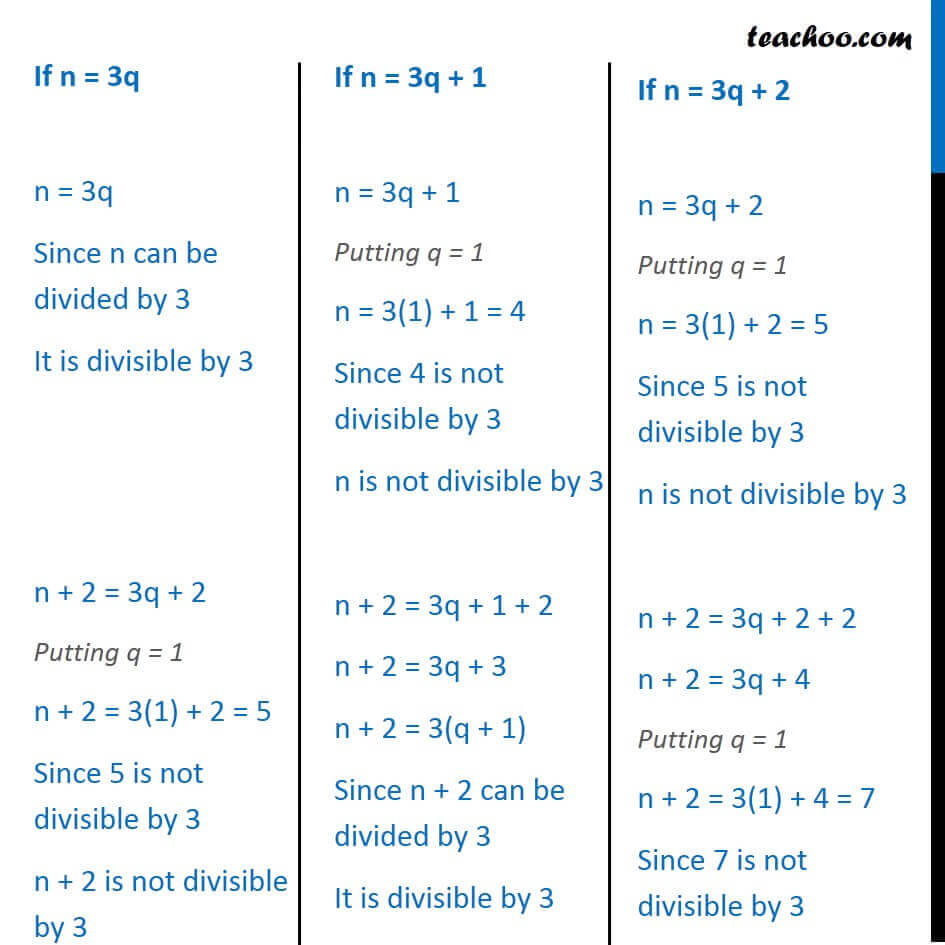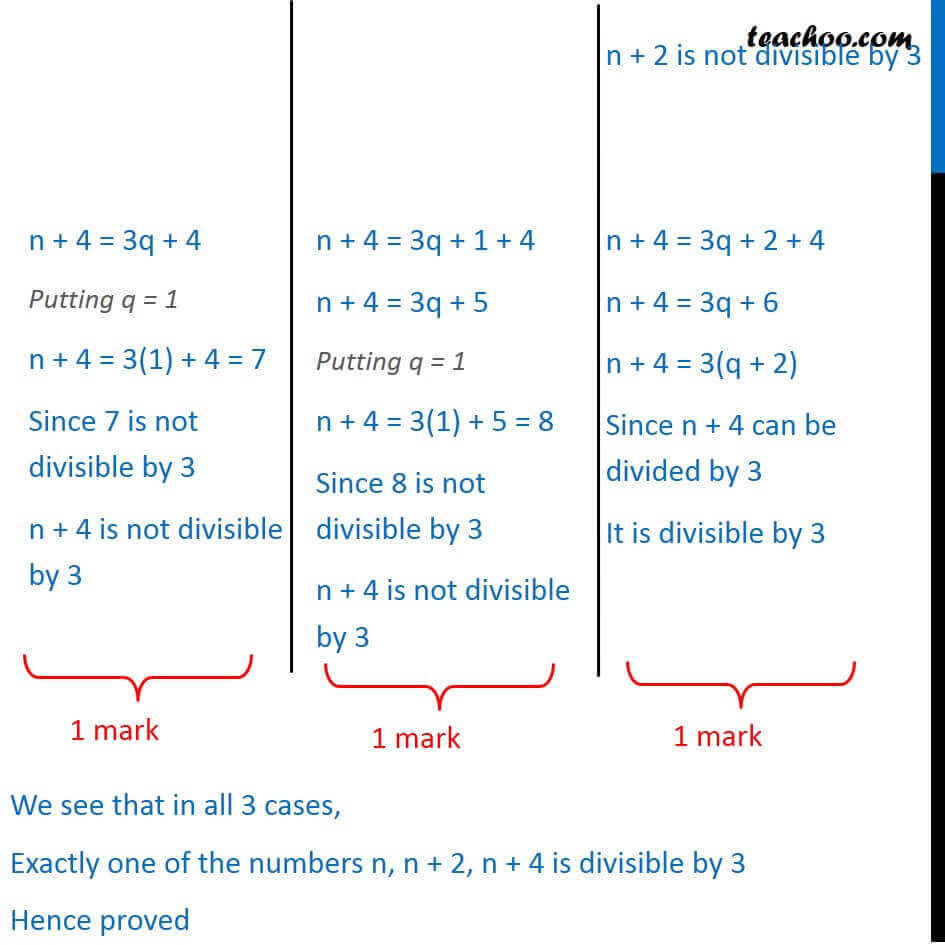CBSE Class 10 Sample Paper for 2018 Boards

Class 10
Solutions of Sample Papers for Class 10 Boards

### Show that exactly one of the numbers n, n + 2 or n + 4 is divisible by 3.

This is a question of CBSE Sample Paper - Class 10 - 2017/18.Learn in your speed, with individual attention - Teachoo Maths 1-on-1 Class

### Transcript

Question 13 Show that exactly one of the numbers n, n + 2 or n + 4 is divisible by 3. Theory As per Euclid’s Division Lemma If a and b are 2 positive integers, then a = bq + r where 0 ≤ r < b If b = 3, a = 3q + r where 0 ≤ r < 3 So, r = 0, 1, 2 ∴ Numbers = 3q + 0, 3q + 1, 3q + 2 Let’s assume n = 3q, 3q + 1, 3q + 2 Now, we check whether n, n + 2, n + 4 is divisible by 3 If n = 3q n = 3q Since n can be divided by 3 It is divisible by 3 n + 2 = 3q + 2 Putting q = 1 n + 2 = 3(1) + 2 = 5 Since 5 is not divisible by 3 n + 2 is not divisible by 3 n + 4 = 3q + 4 Putting q = 1 n + 4 = 3(1) + 4 = 7 Since 7 is not divisible by 3 n + 4 is not divisible by 3 If n = 3q + 1 n = 3q + 1 Putting q = 1 n = 3(1) + 1 = 4 Since 4 is not divisible by 3 n is not divisible by 3 n + 2 = 3q + 1 + 2 n + 2 = 3q + 3 n + 2 = 3(q + 1) Since n + 2 can be divided by 3 It is divisible by 3 n + 4 = 3q + 1 + 4 n + 4 = 3q + 5 Putting q = 1 n + 4 = 3(1) + 5 = 8 Since 8 is not divisible by 3 n + 4 is not divisible by 3 n + 4 = 3q + 1 + 4 n + 4 = 3q + 5 Putting q = 1 n + 4 = 3(1) + 5 = 8 Since 8 is not divisible by 3 n + 4 is not divisible by 3 If n = 3q + 2 n = 3q + 2 Putting q = 1 n = 3(1) + 2 = 5 Since 5 is not divisible by 3 n is not divisible by 3 If n = 3q + 1 n = 3q + 1 Putting q = 1 n = 3(1) + 1 = 4 Since 4 is not divisible by 3 n + 2 is not divisible by 3 n + 4 = 3q + 2 + 4 n + 4 = 3q + 6 n + 4 = 3(q + 2) Since n + 4 can be divided by 3 It is divisible by 3 We see that in all 3 cases, Exactly one of the numbers n, n + 2, n + 4 is divisible by 3 Hence proved